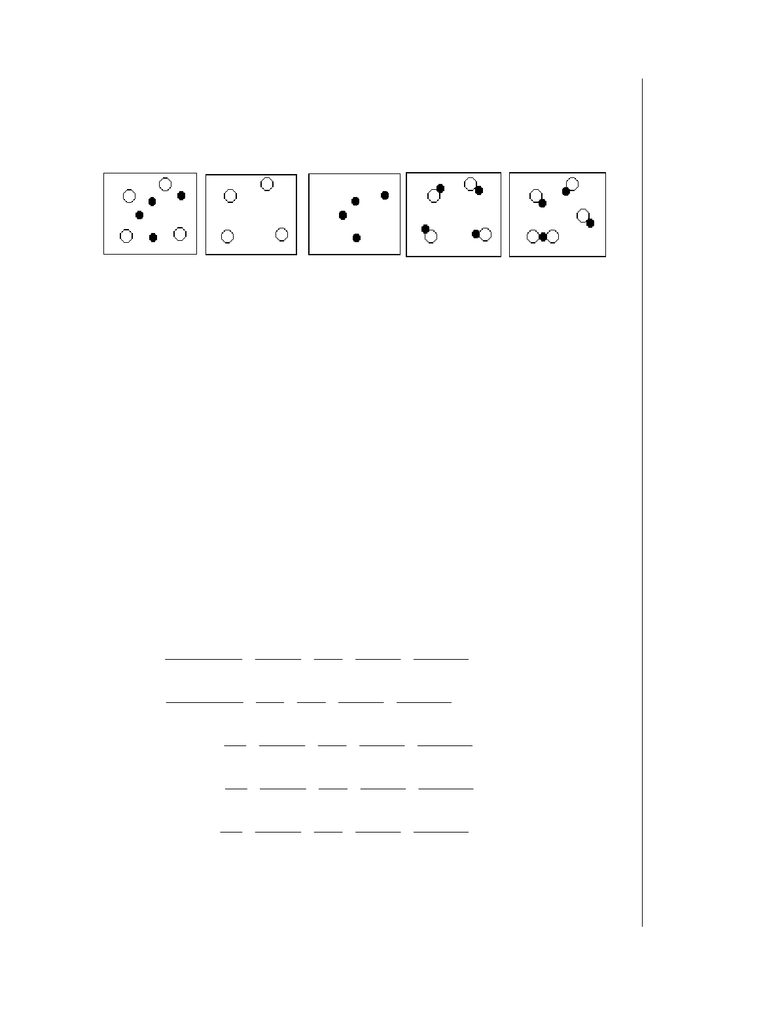Study Guides (380,000)
US (220,000)
ASU (1,000)
CHM (90)
CHM 113 (20)
All (10)
Midterm

# Exam 1Exam

Department
Chemistry
Course Code
CHM 113
Professor
All
Study Guide
Midterm

This preview shows pages 1-2. to view the full 6 pages of the document.Sample Exam 1
Copyright by James P. Birk page 1
Multiple Choice (4 points each): Answer on blue form; be sure to code in your
name and ID.
1. Which of the following figures represent(s) a pure substance? Circles are atoms
and connected circles are molecules.
1 2 3 4 5
a. 1 b. 2
c. 3 d. 4
e. 2, 3, and 4
2. Which of the above figures represent(s) a mixture of elements?
a. 1 b. 4
c. 5 d. 1 and 5
e. 4 and 5
3. Which of the following describes a chemical property of gold?
a. Gold is a yellow metal.
b. Gold is an inert (nonreactive) metal.
c. Gold is a soft metal.
d. Gold is a very dense metal.
e. Gold is a good conductor of heat and electricity.
4. The average velocity of carbon dioxide molecules at 0oC is 3.62 x 104 cm/sec.
Which of the following calculations would convert this value to the velocity in
miles per hour?
a. 1
362 10
254
1
12
1
5280
1
1
3600
4
sec
.
.
sec
xcm
xcm
in xin
ft xft
mi xhr
b. 1
362 10
254 1
12
1
5280
3600
1
4
sec
.
.sec
xcm
xcm xft
in xmi
ft xhr
c. 362 10 1
254
1
12
1
5280
3600
1
4
.sec .
sec
xcm xin
cm xft
in xmi
ft xhr
d. 362 10 254
1
12
1
5280
1
3600
1
4
.sec
.sec
xcm xcm
in xin
ft xft
mi xhr
e. 362 10 1
254
12
1
5280
1
1
3600
4
.sec . sec
xcm xin
cm xin
ft xft
mi xhr
1. e
2. a
3. b
4. c

Only pages 1-2 are available for preview. Some parts have been intentionally blurred.Sample Exam 1
Copyright by James P. Birk page 2
5. Which one of the following lists gives the symbols for the elements phosphorus,
potassium, silver, chlorine, and sulfur, in that order?
a. K, Ag, Po, Cl, S b. P, K, Ag, Cl, S
c. P, Po, Ag, Cl, S d. Ph, K, Ag, S, Cl
e. Ph, Po, Ag, Cl, S
6. Which one of the following statements about the isotopes of a given element is
correct?
a. The atoms have the same number of protons but differing numbers of
neutrons.
b. the atoms have the same number of neutrons but differing numbers of
electrons.
c. The atoms have the same number of electrons but differing numbers of
protons.
d. The atoms have the same number of neutrons but differing numbers of
protons.
e. The atoms have the same number of protons but differing numbers of
electrons.
7. Atom X has 10 protons, 10 neutrons, and 10 electrons. Atom Y has 10 protons,
10 neutrons, and 9 electrons. Atom X and atom Y are
a. isotopes
b. isoelectronic
c. isobars
d. X is the atom and Y the 1+ ion of X
e. both are ions with different charges
8. Calculate the average atomic mass of byzanium, a fictional element, if it has
three isotopes with the following abundances and masses:
45Bz 44.99 amu 30.0%
47Bz 46.99 amu 60.0%
48Bz 48.00 amu 10.0%
a. 45.9 amu b. 46.5 amu
c. 46.7 amu d. 47.0 amu
e. 140 amu
9. Of the following, the only empirical formula is
a. N2F2b. N2F4
c. H2C2d. H2N2O2
e. HNF2
10. A binary compound of bismuth and oxygen contains 89.7% bismuth, Bi. What
is the empirical formula of the compound?
a. Bi2O3b. BiO c. Bi2O
d. BiO2e. Bi3O2
5. b
6. a
7. d
8. b
9. e
10. a
###### You're Reading a Preview

Unlock to view full version